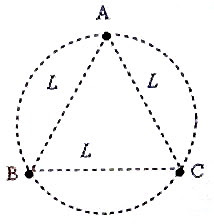## Pages

`“Life is like riding a bicycle.  To keep your balance you must keep moving.”–Albert Einstein`

## Thursday, May 15, 2008

### AP Physics B & C –A Free-Response Practice Question on Gravitation

Working out as many questions as possible will boost your confidence in facing the AP Physics Exam. Constant practice has no substitute and you will definitely be rewarded for your effort. So, start preparing for the AP Physics 2009 Examination without any delay.

The following free response practice question involving gravitation will be useful for both AP Physics B and C aspirants:

Two identical spherical masses M and M initially at rest at very large separation start to move towards each other under their mutual gravitational attraction. Assume that there are no other appreciable fields to affect their motion.

(a) Show that their relative velocity of approach when they are a distance r apart is √(4GM/r) where G is the gravitational constant.

(b) What is the velocity of the centre of mass of this two mass system?

(c) If the two masses are so large that they could eventually form a binary star system, and move along a circle of radius r0 with the masses at opposite ends of a diameter, calculate the orbital velocity of each star in terms of G, M and r0 .(d) If there are three identical masses A, B and C (instead of two) forming a ternary star system with the masses at the vertices of an equilateral triangle of side L and revolving along a circular orbit circumscribing the triangle as shown in the figure, calculate the orbital velocity of each star. [Many ternary star systems have been observed using modern telescopes; but, three identical stars with equal separation arranged on the same circle is rare].

(e) What is the gravitational field at the centroid of the triangle? Justify your answer.

The above question carries 15 points (4+1+3+5+2).

Try to answer this question. I’ll be back with a model answer for you shortly.